n queen topics
Home
Group actions
Search algorithm
Use symmetry, regular NQ problem
Evolution trees
Special properties
Necklaces
Conflict tables
Smallest solutions and greedy sequence
World records
Tables of counting results
Images for n=8
Torus images for n=13
Other pages to the n queens problem
Bibliografy

Other themes
Primes from sums of factors
Primes from wrong binomic formula
Numbers by primes distribution

German

# Conflicts in the n-queens problem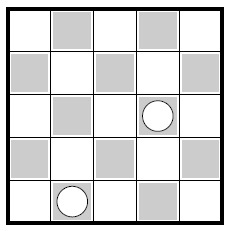A n-queens conflict.

The n-queens problem avoids conflicts between queens, by its proper definition.

There is a reason that I speak of conflicts here, nevertheless: the problem is seen from a different perspective. This perspective unifies the torus problem and the regular problem. The crucial point is: solutions of the regular n-queens problem are seen as torus solutions containing some flaws (or faults). These flaws are exactly the "conflicts" which are in focus here.

To state it a bit more formal: two queens establish a conflict, if they join the same torus diagonal or torus-anti-diagonal. Or, even more formal: if p is a permutation of {0 .. n-1}, i and k different, 0 ≤ i,k < n, and if p(i) - i = p(k) - k (mod n) or p(i) + i = p(k) + k (mod n), then the pair of points (i, p(i)) and (k, p(k)) establishes a conflict for p.

By this, conflicts are defined for all permutations. In the following text, only permutations having a maximum of 2 queens on a (anti-)diagonal are discussed.

With this definition, we can state: a torus n-queens solution is a permutation without conflicts.

# A conflict is rarely coming alone

Solutions to the regular n-queens problem usually have conflicts. Solutions of the torus problems are also solutions to the regular problem, of course. All other solutions have at least one conflict, by definition.

It is surprising that no solutions having only one conflict exist probably. The author thinks he has a proof of this but is not quite sure about it. So, the question is still open a bit. Should someone have another proof or a counterexample, i.e. a solution with only one conflict, he should think of sending it to me.

Torus solutions exist, if and only if n is neither divisible by 2 nor divisible by 3. Looking at the proof, you can see that the first part of the argument is separate for diagonals and for anti-diagonals. Applying this idea, we come to the conclusion that, for n even, there is a conflict on the diagonals, and another on the antidiagonals. That means, there at least two conflicts for n even. But there might exist solutions with only one conflict for odd n. My tests show that there is no such solution, up to the 18 × 18 board.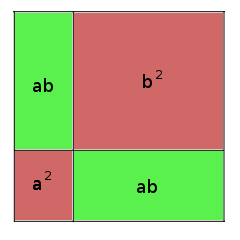A conflict may be resolved; the area for that reminds of the proof of the binomic formula (a + b)² = a² + 2ab + b².

# To resolve conflicts

A torus solution may be shifted in horizontal and vertical direction. This does not work for regular solutions, at least not generally. From the viewpoint of conflicts, the problem is to find a shift combination so that both parts of a conflict are broken by a margin line. After this shift, the queens are "separated", the conflict is resolved.

Every single conflict may be resolved by shifts. I call the set of points leading to a resolved conflict after a shift transfering the point to the origin as separation area of the conflict.

If you paint an image of that situation in the endless, periodic plain, you get something that reminds of the geometric proof of the binomic formula (a + b)² = a² + 2ab + b². The variables a and b correspond to the distance of the queens on the diagonal, and n corresponds to the size of the board and the length of the periodicity. The relation for them is a + b = n. For n queen conflicts, a and b are always different.

As a and b are different, the image contains two square (marked red), and two rectangles which are no squares (marked green). With a shift of the origin to points in the two squares, the conflict remains as conflict, while a shift into the rectangles separates the queens of the conflict. The two rectangles form the separation area.

# Conflict constellationsTwo conflicts, five queens not engaged in conflicts

The set of all queens engaged in a conflict form the conflict constellation of a solution. In other words: a conflict constellation is a special subset of the graph of a permutation. There is a well- defined conflict constellation for every solution of the regular n-queens problem. For torus solutions, the conflict constellation is the empty set.

Usually, the conflict constellation of a solution is a proper subset of all queens. That is shown on the image to the left. But it happens also that all queens are engaged in conflicts, and the conflict constellation is the set of all queens. The first time this happens is on the 4×4 board, and then again on the 12×12 board. No such solutions exist in the range from 5 to 11. For 12, there are four solutions (neglecting rotations and reflections) which are given by images. The conflict lines are contained, and all are broken by the margins, as it is requested for correct solutions.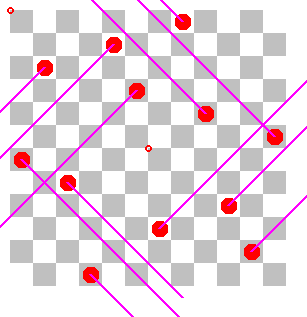All queens are engaged in conflicts - solution 3.

The mapping from n-queens solutions to conflict constellations is neither injective nor surjective, in general. In other words: somtimes, a conflict constellation can be extended (by un-engaged queens) to different solutions, and sometimes, it cannot be extended at all.All queens are engaged in conflicts - solution 13.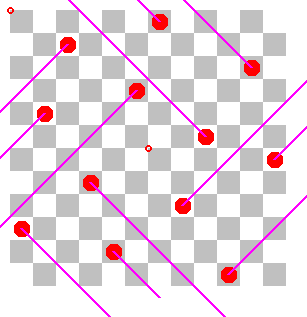All queens are engaged in conflicts - solution 362.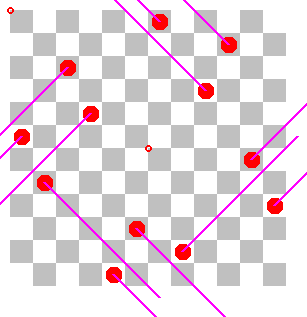All queens are engaged in conflicts - solution 606.

# Values for a classification by conflict constellation

Four values are of interest for a classification of n-queen solutions by their conflict constellations. The third and fourth of these values use a bit of graph theory. The conflict constellation forms a graph in the following way: vertices are the queens, and there is an edge between two vertices if there is a conflict between the two queens, i.e. they are on the same torus diagonal, or on the same torus anti-diagonal.

Due to the fact that there are only two directions of diagonals, this graph has a maximal degree of 2 (degree means the number of edges adjacent to a vertex). A conflict constellation may lead to a cyclic graph. However, no case is known to the author where a cyclic conflict constellation may be extended to a n-queens solution, or even be shifted in a way that all conflicts are resolved. This is another open problem.

We come back to the four measures. These are:

• The number of conflicts.
• The number of queens engaged in a conflict.
• The number of connection components of the conflict graph.
• The maximal size of a connection component of the conflict graph.

Tables of these measures, are given on page conflict tables, for known sizes.

Taking the number of cyclic connection components as fifth value, we have the following relation:

```
Number of engaged queens =    Number of conflicts
+ Number of connection components
- Number of cyclic connection components

```

This relation is not reflected directly in the tables.

Prepared by Matthias Engelhardt

last change: 2015-02-16